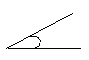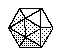Name: ___________________Date:___________________

 Email us to get an instant 20% discount on highly effective K-12 Math & English kwizNET Programs!

### Grade 3 - Mathematics8.19 Geometry Review Test

 Q 1: A line that goes halfway through a circle is araydiameterradius Q 2: An angle that is less than a right angle is ______ angle.Answer: Q 3: A 3 dimensional shape with all faces of the same shape and same size is called aPolygonPentagonPolyhedron Q 4: Area of a rectangle = 2 x area of a triangle =FalseTrue Q 5: What is the shape with 20 faces, each formed by an equilateral triangle?twentyhedronicosahedronhydron Q 6: What is the shape with 12 faces, each formed by an regular pentagon?dodecahedron12hedronsdocahedron Q 7: Two figures that are exactly the same are said to bedifferentcongruentsymmetrical Q 8: An angle that is less than a right angle isangle lessacute anglea right angle Question 9: This question is available to subscribers only! Question 10: This question is available to subscribers only!